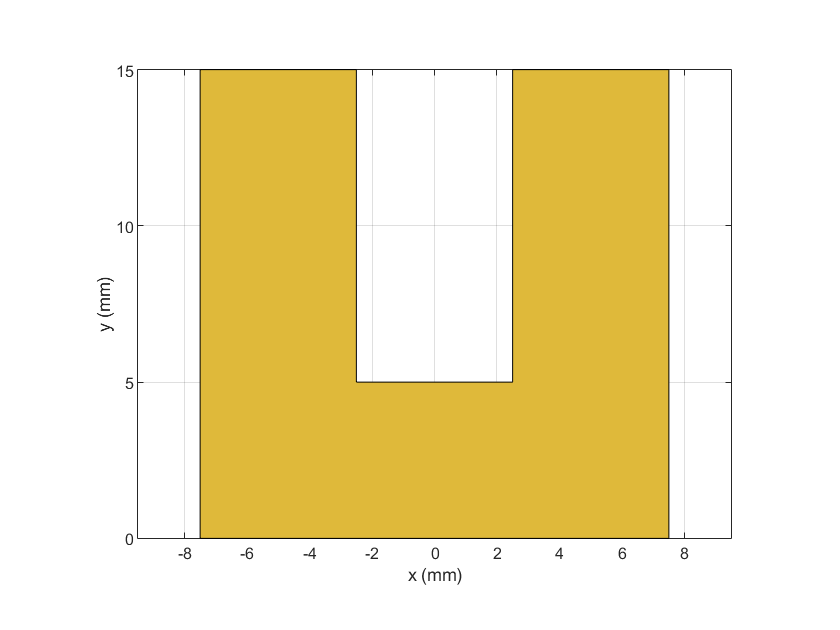# ubendRightAngle

Create right-angle U-bend shape on X-Y plane

## Description

Use the `ubendRightAngle` object to create a right-angle U-bend shape on the X-Y plane.

## Creation

### Syntax

``bend = ubendRightAngle``
``bend = ubendRightAngle(Name=Value)``

### Description

example

````bend = ubendRightAngle` creates a right-angle U-bend shape on the X-Y plane.```
````bend = ubendRightAngle(Name=Value)` sets Properties using one or more name-value arguments. For example, ```ubendRightAngle(ReferencePoint=[1 1])``` creates a right-angle U-bend at the reference point ```[1 1]```. Properties not specified retain their default values.```

## Properties

expand all

Name of the right-angle U-bend, specified as a character vector or a string scalar.

Example: `bend = ubendRightAngle(Name="ubendrightangle1")`

Data Types: `char` | `string`

Reference point of the right-angle U-bend in Cartesian coordinates, specified as a two-element vector.

Example: `bend = ubendRightAngle(ReferencePoint=[1 2])`

Data Types: `double`

Length of the right-angled U-bend in meters, specified as a three-element vector of positive elements.

Example: `bend = ubendRightAngle(Length=[0.0250 0.0150 0.0250])`

Data Types: `double`

Width of the right-angle U-bend in meters, specified as a three-element vector of positive values.

Example: `bend = ubendRightAngle(Width=[0.0150 0.0150 0.0010])`

Data Types: `double`

## Object Functions

 `add` Boolean unite operation on two RF PCB shapes `and` Shape1 & Shape2 for RF PCB shapes `area` Calculate area of RF PCB shape in square meters `intersect` Boolean intersection operation on two RF PCB shapes `mesh` Change and view mesh properties of metal or dielectric in PCB component `minus` Shape1 - Shape2 for RF PCB shapes `plus` Shape1 + Shape2 for RF PCB shapes `rotate` Rotate RF PCB shape about defined axis `rotateX` Rotate RF PCB shape about x-axis `rotateY` Rotate RF PCB shape about y-axis and angle `rotateZ` Rotate RF PCB shape about z-axis `subtract` Boolean subtraction operation on two RF PCB shapes `scale` Change size of RF PCB shape by fixed amount `show` Display PCB component structure or PCB shape `translate` Move RF PCB shape to new location

## Examples

collapse all

Create a right-angle U-bend with default properties.

`bend = ubendRightAngle`
```bend = ubendRightAngle with properties: Name: 'myRightAngleubend' ReferencePoint: [0 0] Length: [0.0150 0.0050 0.0150] Width: [0.0050 0.0050 0.0050] ```

View the shape.

`show(bend)`## Version History

Introduced in R2021b